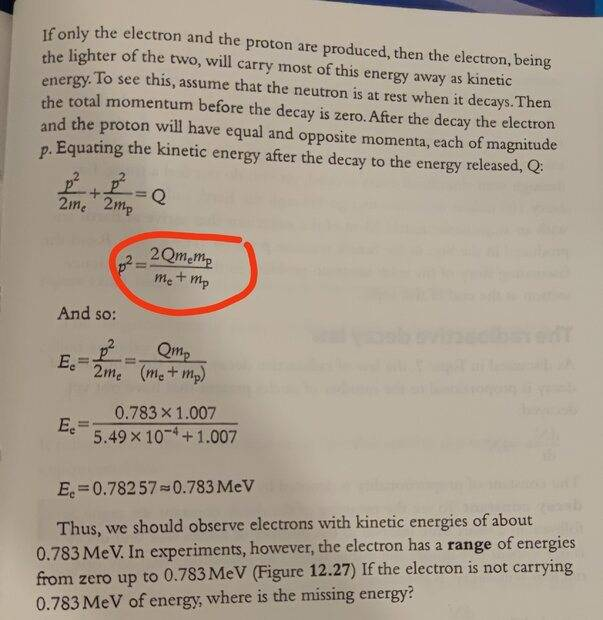# Electron energy derivation

Woopa
Homework Statement:
Derive the equation
Relevant Equations:
E=P^2/2mI am having trouble understanding this derivation and need some guidance.

1) I tried solving the algebra from the first equation to the second equation circled in red. Can someone please help with what algebra steps, I cannot solve to the circled solution.

2) What does Ee stand for? Is it Total energy of the electron?

3) Where did the value 5.49x10-4 come from? This does not appear to be the mass of the electron.

Staff Emeritus
Homework Helper
Gold Member

2) Kinetic energy of the electron

3) it is the electron mass’ numerical value in the atomic mass unit u (which also the proton mass is given in). Units are important.

Mentor
Homework Statement:: Derive the equation
Relevant Equations:: E=P^2/2m

1) I tried solving the algebra from the first equation to the second equation circled in red. Can someone please help with what algebra steps, I cannot solve to the circled solution.
What did you try? Hint: $$\frac{A}{B} + \frac{A}{C} = A(\frac{1}{B} + \frac{1}{C})$$

Hint: Find a common denominator to express that sum of fractions as a single fraction.

•